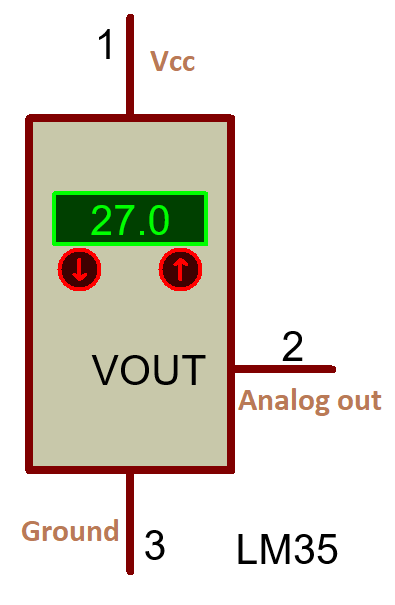## LM35 -Temperature Sensor

It is an analog temperature sensor. It is also called a linear device that means output voltage proportional to the temperature. These are small in size and consumes very little current up to  60 uA only and it provides highly readable readings. There is no need for large mathematical manipulations. It is available  in hermetic TO-46 transistor packages

Model number: LM35

Other names: Heat sensing device.

Other related devices: LM35DT, DHT11, DHT22,

DATA SHEETLM35LM35

## Pin Configuration

 Pin number Pin name Description 1 Ground Ground connection 2 Vout Analog output 3 Vcc 5v (positive pin)

## Technical Specifications

 Module LM35 Type Analog sensor Operating Voltage 4v- 30v Operating Temperature -55  ͦC  to  150  ͦC Accuracy 0.5  ͦC    at 25  ͦC Current drain 60-μA Self-Heating 0.08  C   in  air Temperature Scale factor + 10.0 mV/˚C package Through-hole PCB mount TO-46 Total pins 3

## How to Convert analog Readings into Temperature

We know that Arduino has a 10-bit ADC so the maximum rating for 5v is 1023.

• The maximum out voltage for LM35 at 150  ͦ C is 1500mv.
• For 5v the we have 1023 readings ,so for 1500mv (1.5V) = (1.5x 1023)/5 = 306.9
• At 150  ͦ C, LM35 output maximum voltage 1500mv the obtain maximum reading is 306.9.
• At 150  ͦ C  output readings 306.9, then for 1  ͦ C  the readings become 306.9 / 150 = 2.046, this is the value used to convert LM35 analog out readings into   ͦ C.
Temparature  = anlogRead(temp)/ 2.046    ( ͦ C )

## Hardware requirement

It is very easy to interface because LM35 no need any an external circuit like Thermistor

We know that LM35 has 3 pins (VCC, Vout, Gnd)

 LM35 ARDUINO VCC 5V Vout(analog output) A0 GND GNDLM35_Arduino_InterfaceLM35_Arduino_Interface

Circuit explanation

LM35 has 3 pins Vcc, Gnd, OUT.

Vcc is power supply positive pin, Gnd is ground pin , OUT is sensor Analog output pin.

Vcc pin is connected to 5v Arduino pin, Gnd  is connected to GND of Arduino pin, OUT pin is connected to A0 pin of Arduino.

Note: sensor output is the analog signal, so we have to connect to ADC Channel-0 (A0).

Arduino code for LM35

## Code ExplanationBefore write the code make the hardware connections and study how to convert analog readings to temperature.Code starts with “LiquidCrystal.h” declaration, it contains the LCD functionalities. #include<LiquidCrystal.h&ht; Next we have to declare variables for the hardware pin connections such as sensor output  temp=A0, and for LCD pins lcd(2,3,4,5,6,7); #include<LiquidCrystal.h&ht; LiquidCrystal lcd(2,3,4,5,6,7); int temp=A0; int Temperature; We declare the variable “Temperature” that stores the sensor readings. Here the setup() function has used for one time  executed instructions, in the setup() function we declare the sensor output as input signal by using “pinMode”  keyword and LCD initialized using lcd.began(16,2) ,lcd.clear() is used to clear the LCD, lcd.print() is used to print the data on LCD. pinMode(temp,A0); lcd.begin(16,2); lcd.clear(); lcd.print("Temp:"); next, we go for the loop() function it is also called an infinite loop used for continuous execution of instructions, using the loop() function we are continuously monitoring the sensor output if there is any changes we can get the updated data.“analogRead” keyword is used to  get the sensor output Temperature= analogRead(A0)/2.046; and lcd.setCursor() is used to set the data position of LCD, where you want to print the data on LCD first row, second row or any column.Syntax: lcd.setCursor(column,row)Example:  lcd.setCursor(5,0);  à indicates the 5th column ,first row. Temperature= analogRead(A0)/2.046; lcd.setCursor(5,0); lcd.print(Temperature); lcd.setCursor(8,0); lcd.print("`C"); here this code tested and excecuted.

Applications

• Microwave Oven
• Air conditioner
• Automobiles
• Power supply
• Battery management

Datasheet

## Other Articles

>>>HOME-TechnoElectronics44>>>

1.1.2.1.